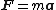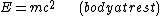# rest mass

(redirected from Invariant mass)
Also found in: Dictionary, Thesaurus, Medical, Wikipedia.
Related to Invariant mass: rest mass, relativistic mass

## Rest mass

A constant intrinsic to a body which determines its inertial and energy-momentum properties. It is a fundamental concept of special relativity, and in particular it determines the internal energy content of a body. It is the same as the inertial mass of classical mechanics. According to the principle of equivalence, the basic physical principle of general relativity, the inertial mass of a body is also equal to its gravitational mass. See Classical mechanics, Gravitation, Relativity

The rest mass or inertial mass of a body, m, is a measure of its resistance to being accelerated at a by a force F ; in classical mechanics the relation between inertial mass, acceleration, and force is given by Newton's law, Eq. (1).

(1)In special relativity Newton's law holds exactly only in the body's rest frame, that is, the frame in which the body is instantaneously at rest. See Newton's laws of motion

Associated with the rest mass of a body, there is an internal or rest energy. In the system where the body is at rest, the energy of the body is given

(2)by Eq. (2).

The experimental realization of the interconversion of mass and energy is accomplished in the reactions of nuclei and elementary particles. In particular, the energy source of nuclear bombs and nuclear fission reactors is a small decrease in the total mass of the interacting nuclei, which gives rise to a large energy release because of the large numerical value of c2. See Elementary particle, Nuclear fission

## rest mass

The mass of an elementary particle, etc., when at rest. According to the special theory of relativity, a body's mass increases as its speed increases. This is noticeable only at speeds close to the speed of light. Rest mass is often expressed in MeV (megaelectronvolts).

## rest mass

[′rest ‚mas]
(relativity)
The mass of a particle in a Lorentz reference frame in which it is at rest.
References in periodicals archive ?
These analogies, in turn, uncover the Relativistic Invariant Mass Paradox in Section 5, the physical significance of which is illustrated in Section 6 in the frame of Einstein's special theory of relativity.
be an isolated Einsteinian system of N noninteracting material particles the k-th particle of which has invariant mass [m.
is the relativistic invariant mass of the Einsteinian system S, supposed concentrated at the relativistic center of mass of S (calculated in [1, Chap.
The Einsteinian resultant mass equation (12) presents a Paradox, called the Relativistic Invariant Mass Paradox, since, in general, this equation implies the inequality

Site: Follow: Share:
Open / Close